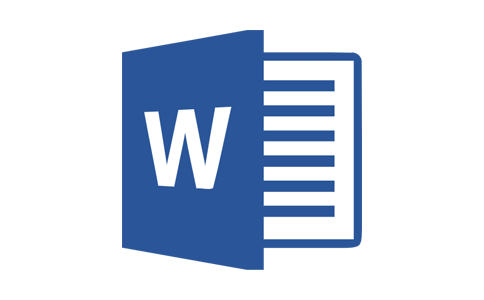# 小学六年级数学图形计算公式大全1、正方形C周长S面积a边长周长=边长×4C=4a面积=边长×边长S=a×a

2、正方体V:体积a:棱长表面积=棱长×棱长×6S表=a×a×6体积=棱长×棱长×棱长V=a×a×a

3、长方形

C周长S面积a边长

C=2(a+b)

S=ab

4、长方体

V:体积s:面积a:长b:宽h:高

(1)表面积(长×宽+长×高+宽×高)×2

S=2(ab+ah+bh)

(2)体积=长×宽×高

V=abh

5三角形

s面积a底h高

s=ah÷2

6平行四边形

s面积a底h高

s=ah

7梯形

s面积a上底b下底h高

s=(a+b)×h÷2

8圆形

S面积C周长∏d=直径r=半径

(1)周长=直径×∏=2×∏×半径

C=∏d=2∏r

(2)面积=半径×半径×∏

9圆柱体

v:体积h:高s;底面积r:底面半径c:底面周长

(1)侧面积=底面周长×高

(2)表面积=侧面积+底面积×2

(3)体积=底面积×高

(4)体积=侧面积÷2×半径

10圆锥体

v:体积h:高s;底面积r:底面半径相关文章栏目最新随机推荐